×

Search anything:

# Learn to use TPOT: An AutoML Tool

#### Machine Learning (ML)Indian Technical Authorship Contest starts on 1st July 2023. Stay tuned.

Reading time: 30 minutes | Coding time: 10 minutes

In this article, I will share some of my insights based on TPOT (Tree Base Pipeline Optimization Tool). I will explain this tool through a dataset I used.

## What is TPOT?

TPOT stands for Tree Base Pipeline Optimization Tool. It is used to solve or give a idea on machine learning problems. It helps us to explore some of pipeline confiuration that we did not consider earlier for our model. It helps us to find out best algorithm for the problem we are working on. We feed the data which is the train input and train output. It analyzes the data and tell us the best machine learning model for the purpose.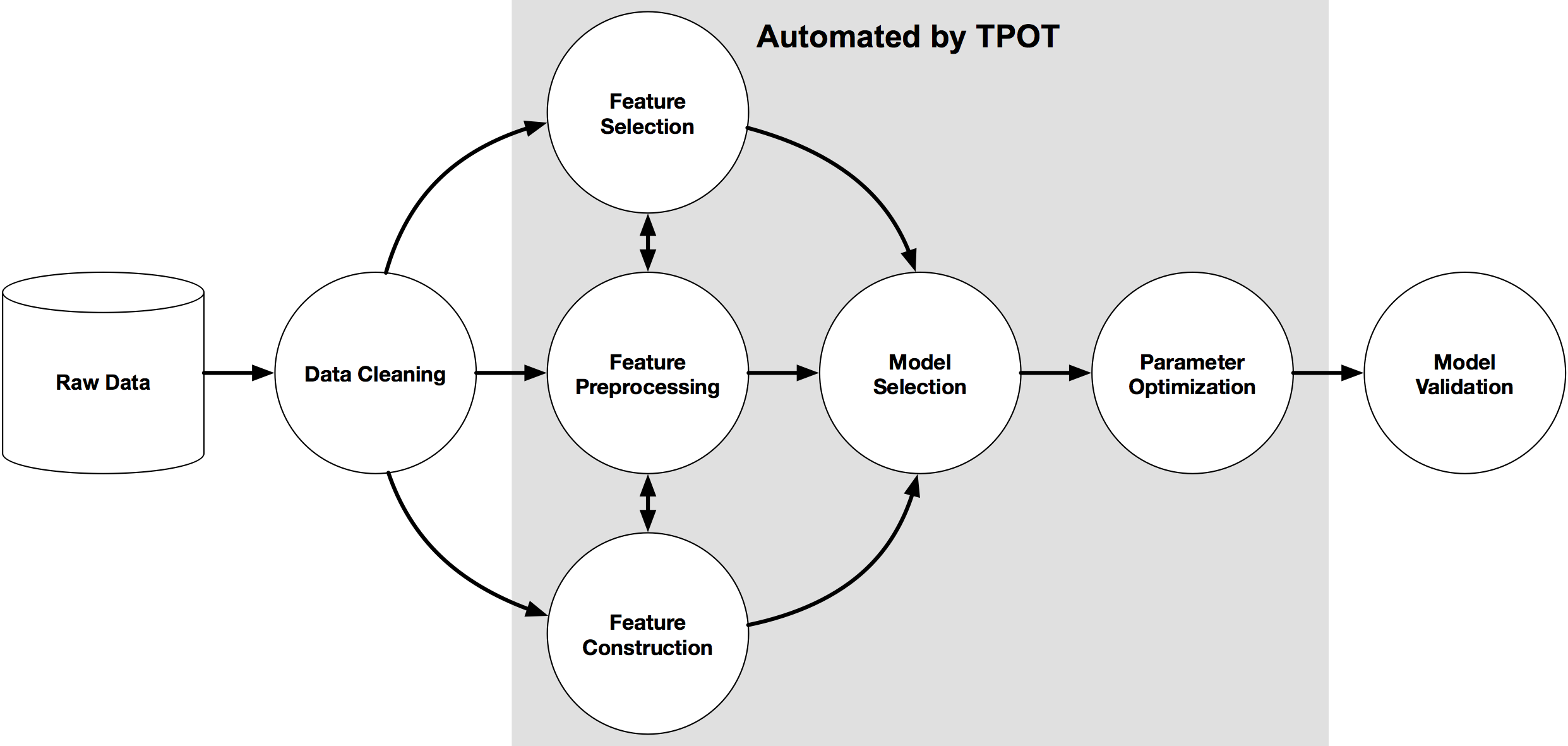TPOT is open source and is a part of scikit learn library. It can be used for both regression and classification models. Implementation and loading of library is different for each.

## Working of TPOT (Using a dataset)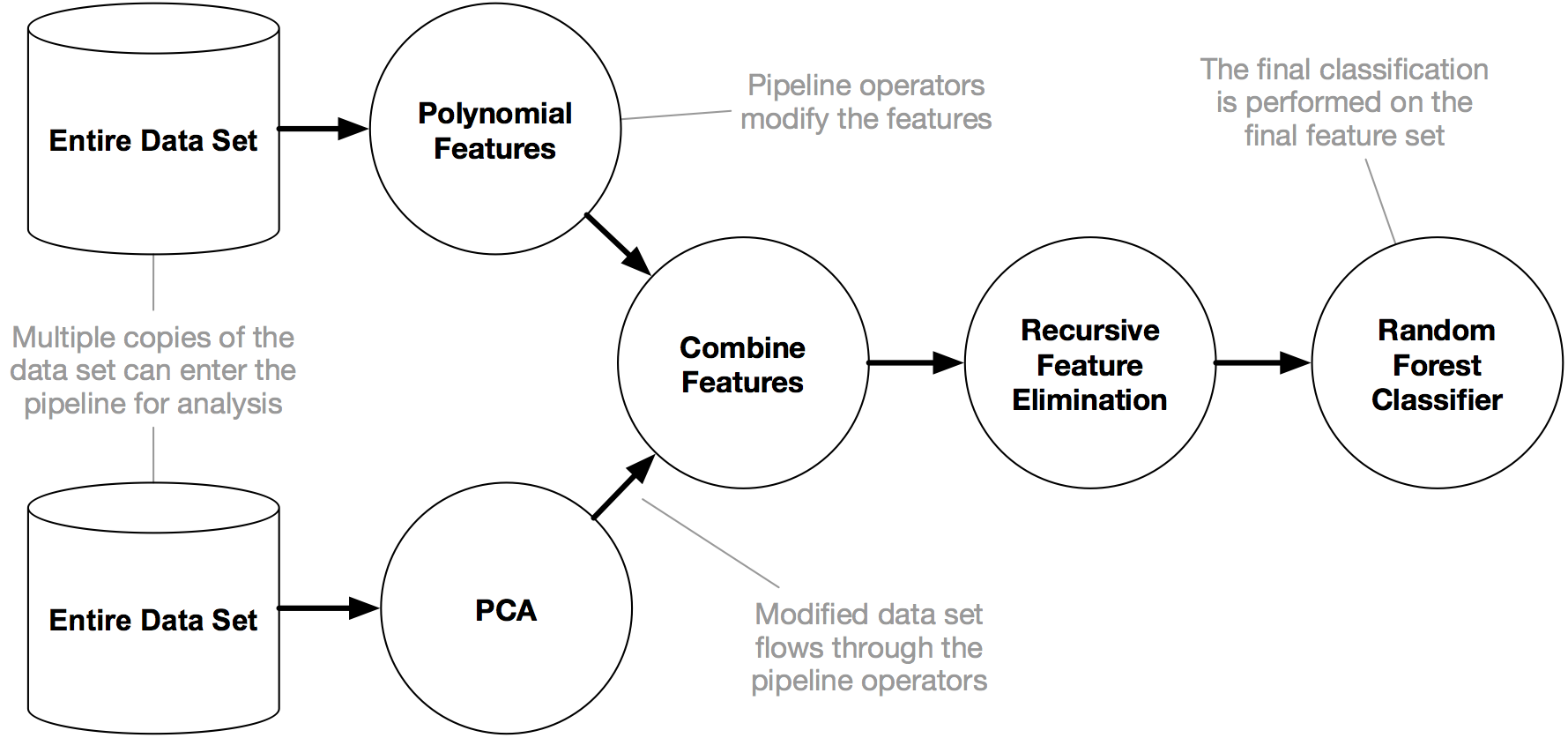It is like a search algorithm which usually searches best algorithm for the purpose. The final search results basically depends on performance means which algorithm providing greater accuracy than other algorithms.It also tunes some hyperparametres for better performances and evaluation. So it cannot be considered as a random search algorithm.

Now generally there are two types of TPOT:

• TPOT classifier
• TPOT regressor.

We will study working of each using a dataset.

## TPOT Classifier

For the purpose we have taken a dataset of a bank data which contains information about their customers.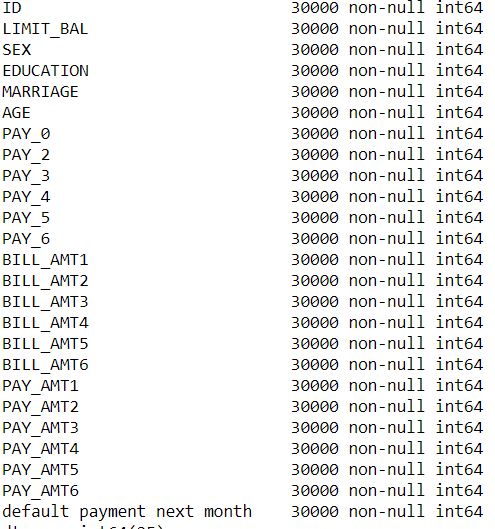Data cleaning was performed to get the data into required form. After performing several function we get the data into required form.

Response Variable: default payment next month
Exploratory Variable: ID, Marriage, Age, Bill_AMT1...Bill_AMT5

``````train=df.drop('default payment next month',axis=1)
test=df['default payment next month']

from sklearn.preprocessing import StandardScaler
sc=StandardScaler()
train=sc.fit_transform(train)

from sklearn import model_selection
x_train,x_test,y_train,y_test=model_selection.train_test_split(train,test)
``````

### Implementing TPOT Classifier

``````from tpot import TPOTClassifier
from sklearn.metrics import roc_auc_score
``````

The default TPOTClassifier arguments:

``````generations=100,
population_size=100,
offspring_size=None  # Jeff notes this gets set to population_size
mutation_rate=0.9,
crossover_rate=0.1,
scoring="Accuracy",  # for Classification
cv=5,
subsample=1.0,
n_jobs=1,
max_time_mins=None,
max_eval_time_mins=5,
random_state=None,
config_dict=None,
warm_start=False,
memory=None,
periodic_checkpoint_folder=None,
early_stop=None
verbosity=0
disable_update_check=False
``````

Our Model:

``````pot = TPOTClassifier(
generations=5,
population_size=20,
verbosity=2,
scoring='roc_auc',
random_state=42,
disable_update_check=True,
config_dict='TPOT light'
)
tpot.fit(x_train, y_train)
``````

After running the model we get the result: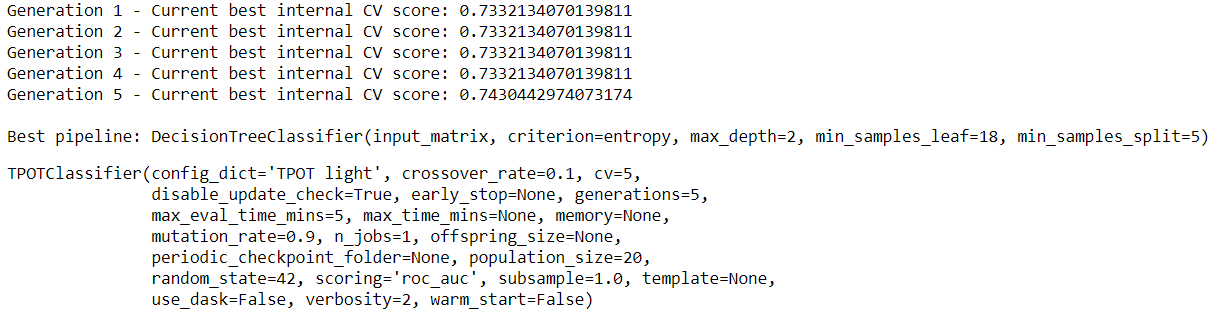``````tpot_auc_score = roc_auc_score(y_test, tpot.predict_proba(x_test)[:, 1])
print(f'\nAUC score: {tpot_auc_score:.4f}')
``````

Output:

``````0.660
``````

To know the best model:

``````print('\nBest pipeline steps:', end='\n')
for idx, (name, transform) in enumerate(tpot.fitted_pipeline_.steps, start=1):
print(f'{idx}. {transform}')
``````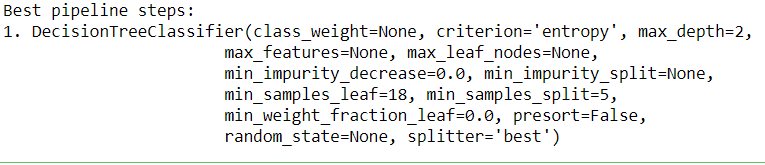Further you can perform other algorithms like GridSearchCV to tune the hyperparametres.

Results we got after using the algorithm told by tpot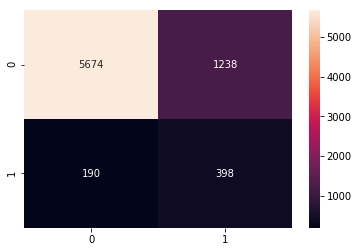## TPOT Regressor

For this purpose we considered a dataset from RBI website.
We performed the data cleaning part and recieve the data in required form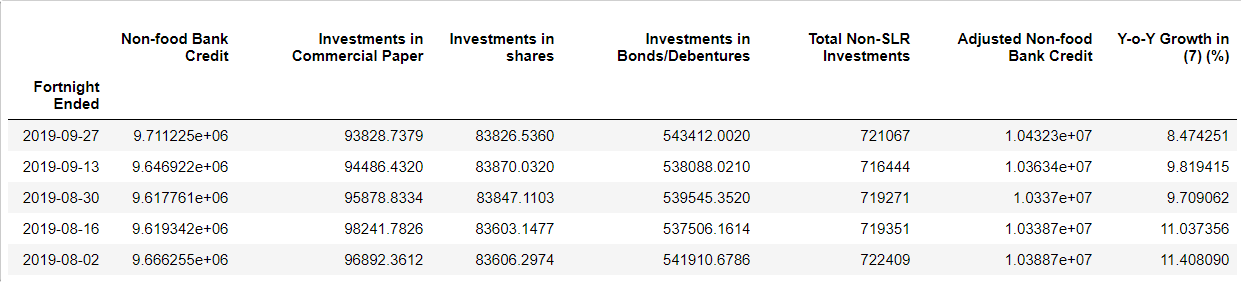Response Variable: Growth

``````from sklearn.preprocessing import StandardScaler
sc=StandardScaler()
df=sc.fit_transform(df)

y=df[' Y-o-Y Growth in (7) (%)']
x=df.drop(' Y-o-Y Growth in (7) (%)',axis=1)

from sklearn import model_selection
x_train,x_test,y_train,y_test=model_selection.train_test_split(x,y)
``````

Correlation of variable with response variable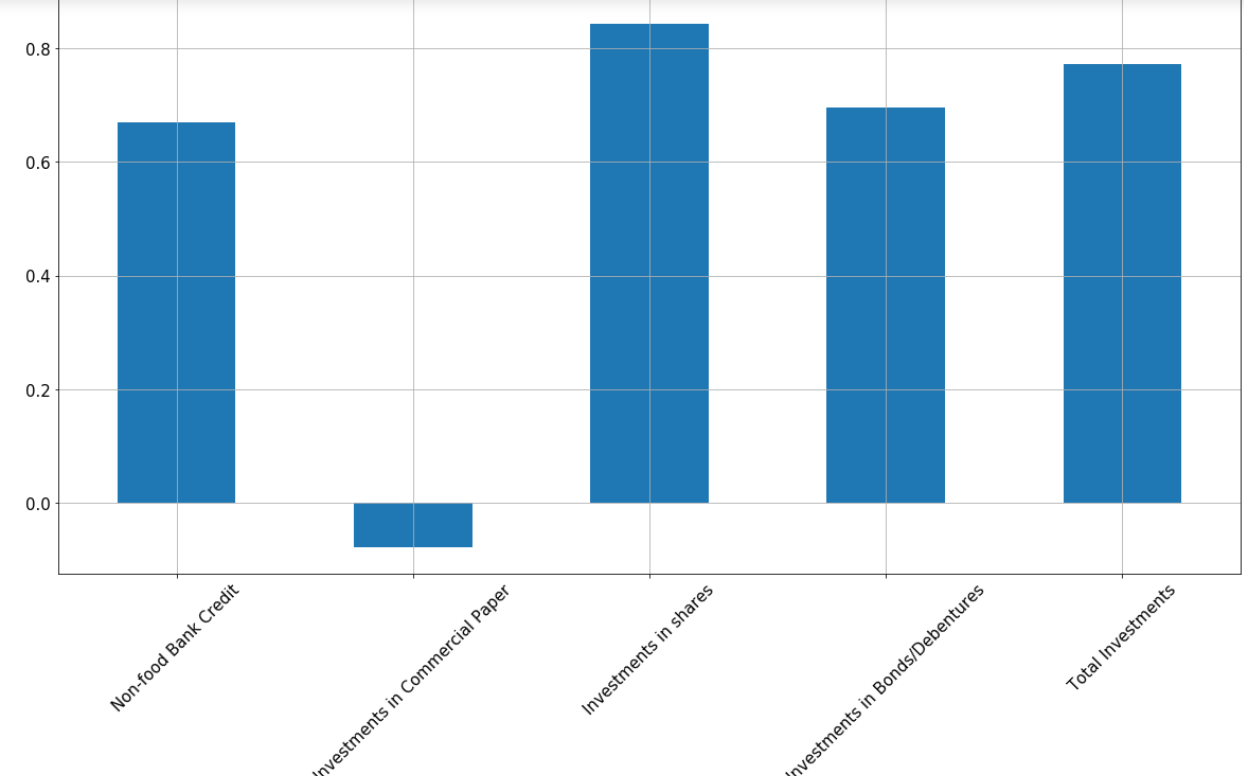### Model Implementaion

``````from tpot import TPOTRegressor
from sklearn.metrics import roc_auc_score

tpot = TPOTRegressor(
generations=5,
population_size=50,
verbosity=2,
)
tpot.fit(x_train, y_train)
``````

After running the model we get the results: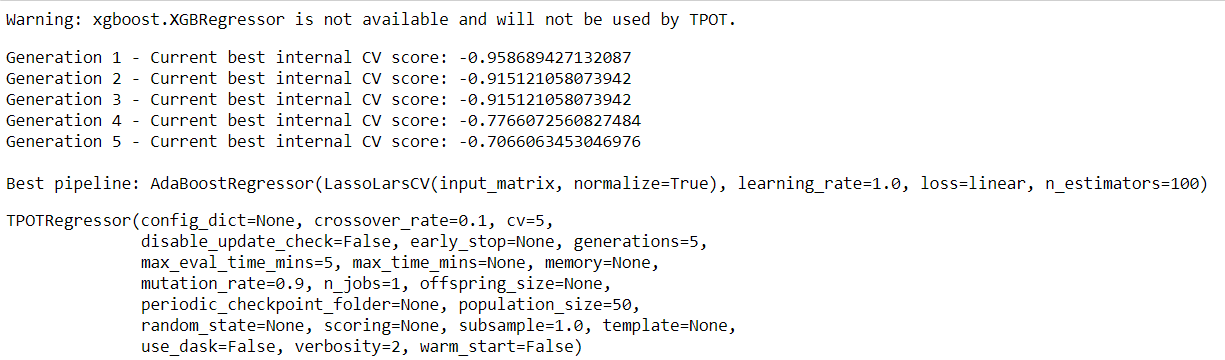After using the algorithm told by TPOT we got excellent results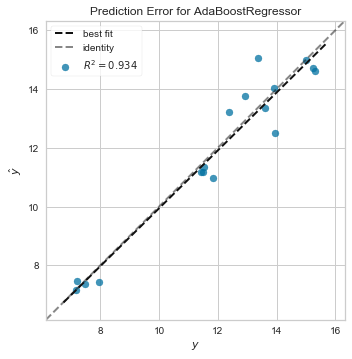There are some parametres on which tpot determine number of pipeline to be searched

• generations: int, optional (default: 100)
Number of iterations to the run pipeline optimization process. Generally, TPOT will work better when you give it more generations(and therefore time) to optimize the pipeline. TPOT will evaluate POPULATION_SIZE + GENERATIONS x OFFSPRING_SIZE pipelines in total (emphasis mine).

• population_size: int, optional (default: 100)
Number of individuals to retain in the GP population every generation.
Generally, TPOT will work better when you give it more individuals (and therefore time) to optimize the pipeline.

• offspring_size: int, optional (default: None)
Number of offspring to produce in each GP generation. By default, offspring_size = population_size.

Algoriths included in latest tpot update:

``````‘sklearn.naive_bayes.BernoulliNB’: { ‘alpha’: [1e-3, 1e-2, 1e-1, 1., 10., 100.], ‘fit_prior’: [True, False] },
‘sklearn.naive_bayes.MultinomialNB’: { ‘alpha’: [1e-3, 1e-2, 1e-1, 1., 10., 100.], ‘fit_prior’: [True, False] },
‘sklearn.tree.DecisionTreeClassifier’: { ‘criterion’: [“gini”, “entropy”], ‘max_depth’: range(1, 11), ‘min_samples_split’: range(2, 21), ‘min_samples_leaf’: range(1, 21) },
‘sklearn.ensemble.ExtraTreesClassifier’: { ‘n_estimators’: , ‘criterion’: [“gini”, “entropy”], ‘max_features’: np.arange(0.05, 1.01, 0.05), ‘min_samples_split’: range(2, 21), ‘min_samples_leaf’: range(1, 21), ‘bootstrap’: [True, False] },
‘sklearn.ensemble.RandomForestClassifier’: { ‘n_estimators’: , ‘criterion’: [“gini”, “entropy”], ‘max_features’: np.arange(0.05, 1.01, 0.05), ‘min_samples_split’: range(2, 21), ‘min_samples_leaf’: range(1, 21), ‘bootstrap’: [True, False] },
‘sklearn.ensemble.GradientBoostingClassifier’: { ‘n_estimators’: , ‘learning_rate’: [1e-3, 1e-2, 1e-1, 0.5, 1.], ‘max_depth’: range(1, 11), ‘min_samples_split’: range(2, 21), ‘min_samples_leaf’: range(1, 21), ‘subsample’: np.arange(0.05, 1.01, 0.05), ‘max_features’: np.arange(0.05, 1.01, 0.05) },
‘sklearn.neighbors.KNeighborsClassifier’: { ‘n_neighbors’: range(1, 101), ‘weights’: [“uniform”, “distance”], ‘p’: [1, 2] },
‘sklearn.svm.LinearSVC’: { ‘penalty’: [“l1”, “l2”], ‘loss’: [“hinge”, “squared_hinge”], ‘dual’: [True, False], ‘tol’: [1e-5, 1e-4, 1e-3, 1e-2, 1e-1], ‘C’: [1e-4, 1e-3, 1e-2, 1e-1, 0.5, 1., 5., 10., 15., 20., 25.] },
‘sklearn.linear_model.LogisticRegression’: { ‘penalty’: [“l1”, “l2”], ‘C’: [1e-4, 1e-3, 1e-2, 1e-1, 0.5, 1., 5., 10., 15., 20., 25.], ‘dual’: [True, False] },
‘xgboost.XGBClassifier’: { ‘n_estimators’: , ‘max_depth’: range(1, 11), ‘learning_rate’: [1e-3, 1e-2, 1e-1, 0.5, 1.], ‘subsample’: np.arange(0.05, 1.01, 0.05), ‘min_child_weight’: range(1, 21), ‘nthread’:  }
``````

# Limitation

• TPOT sometimes take very long for the search of algorithm. Since it searches all algorithm, apply them on data we provided which can take long time. If we provide data without any preprocessing steps, it would take even more time as it first implement those steps and then apply the algorithms.

• In some cases TPOT shows different results for same data provided. This happens when we work on complex dataset#### Harsh Bansal

Machine Learning Developer, Intern at OpenGenus | Bachelor of Technology Student in IT at Northern India Engineering College